This vignette showcases the functions `regressionImp()` and `rangerImpute()`, which can both be used to generate imputations for several variables in a dataset using a formua interface.

### Data

For data, a subset of `sleep` is used. The columns have been selected deliberately to include some interactions between the missing values.

``````library(VIM)
library(magrittr)
dataset <- sleep[, c("Dream", "NonD", "BodyWgt", "Span")]
dataset\$BodyWgt <- log(dataset\$BodyWgt)
dataset\$Span <- log(dataset\$Span)
aggr(dataset)
``````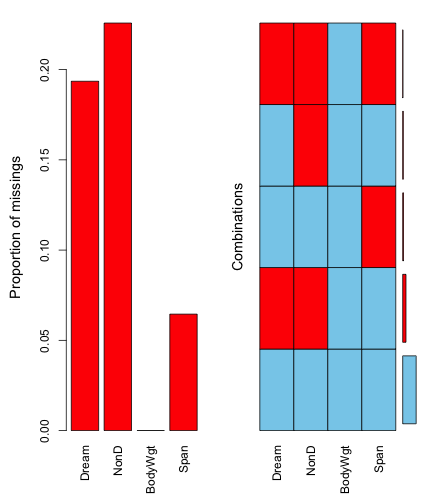``````str(dataset)
#> 'data.frame':    62 obs. of  4 variables:
#>  \$ Dream  : num  NA 2 NA NA 1.8 0.7 3.9 1 3.6 1.4 ...
#>  \$ NonD   : num  NA 6.3 NA NA 2.1 9.1 15.8 5.2 10.9 8.3 ...
#>  \$ BodyWgt: num  8.803 0 1.2194 -0.0834 7.8427 ...
#>  \$ Span   : num  3.65 1.5 2.64 NA 4.23 ...
``````

### Imputation

In order to invoke the imputation methods, a formula is used to specify which variables are to be estimated and which variables should be used as regressors. We will start by imputing `NonD` based in `BodyWgt` and `Span`.

``````imp_regression <- regressionImp(NonD ~ BodyWgt + Span, dataset)
#> There still missing values in variable NonD . Probably due to missing values in the regressors.
imp_ranger <- rangerImpute(NonD ~ BodyWgt + Span, dataset)
aggr(imp_regression, delimiter = "_imp")
``````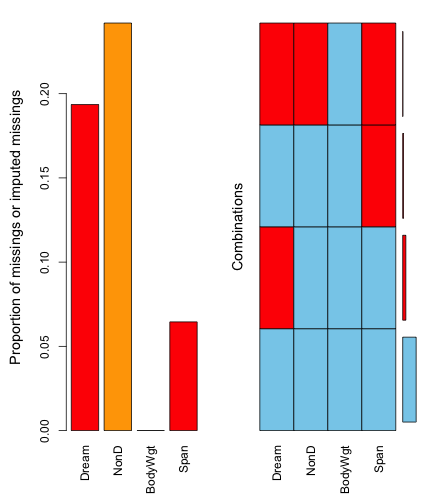We can see that there are still missings in `NonD` for all observations where `Span` is unobserved. This is because the regression model could not be applied to those observations. The same is true for the values imputed via `rangerImpute()`.

### Diagnosing the results

As we can see in the next two plots, the correlation structure of `NonD` and `BodyWgt` is preserved by both imputation methods. In the case of `regressionImp()` all imputed values almost follow a straight line. This suggests that the variable `Span` had little to no effect on the model.

``````imp_regression[, c("NonD", "BodyWgt", "NonD_imp")] %>%
marginplot(delimiter = "_imp")
``````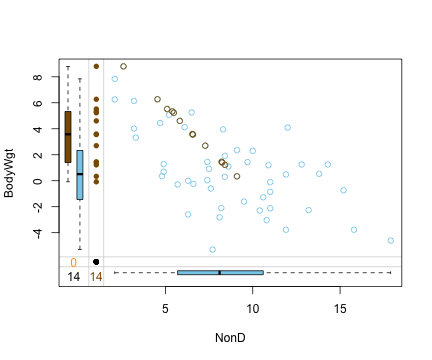For `rangerImpute()` on the other hand, `Span` played an important role in the generation of the imputed values.

``````imp_ranger[, c("NonD", "BodyWgt", "NonD_imp")] %>%
marginplot(delimiter = "_imp")
``````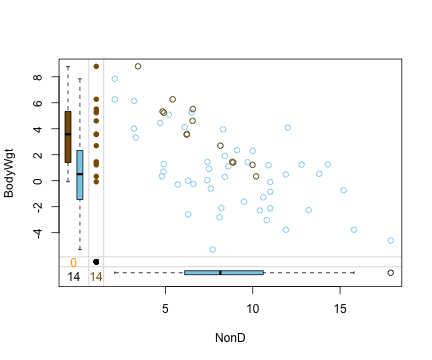``````imp_ranger[, c("NonD", "Span", "NonD_imp")] %>%
marginplot(delimiter = "_imp")
``````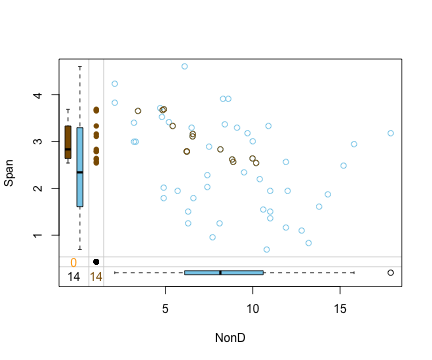### Imputing multiple variables

To impute several variables at once, the formula in `rangerImpute()` and `regressionImp()` can be specified with more than one column name in the left hand side.

``````imp_regression <- regressionImp(Dream + NonD ~ BodyWgt + Span, dataset)
#> There still missing values in variable Dream . Probably due to missing values in the regressors.
#> There still missing values in variable NonD . Probably due to missing values in the regressors.
imp_ranger <- rangerImpute(Dream + NonD ~ BodyWgt + Span, dataset)
aggr(imp_regression, delimiter = "_imp")
``````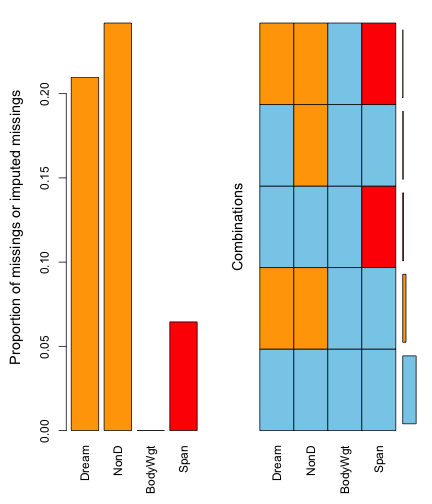Again, there are missings left for both `Dream` and `NonD`.